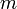# FAQ: What does it mean for a non-square matrix to be full rank?

(diff) ← Older revision | Latest revision (diff) | Newer revision → (diff)
For a non-square matrix withrows andcolumns, it will always be the case that either the rows or columns (whichever is larger in number) are linearly dependent. Hence when we say that a non-square matrix is full rank, we mean that the row and column rank are as high as possible, given the shape of the matrix. So if there are more rows than columns (), then the matrix is full rank if the matrix is full column rank.